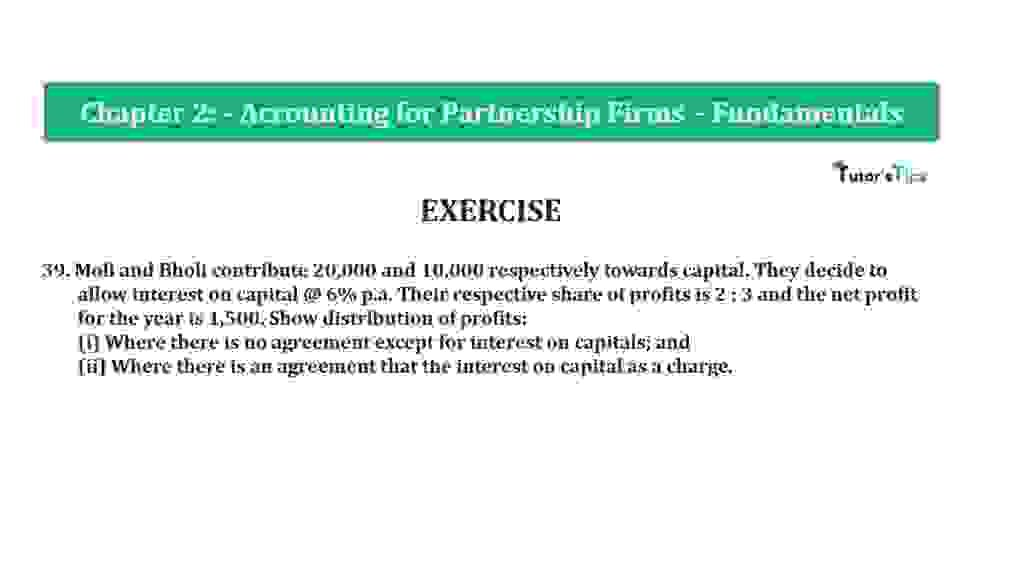# Question 39 Chapter 2 of +2-A – T.S. Grewal 12 Class Part – A Vol. 1Question 39 Chapter 2 of +2-A

39. Moli and Bholi contribute 20,000 and 10,000 respectively towards the capital. They decide to
allow interest on capital @ 6% p.a. Their respective share of profits is 2 : 3 and the net profit
for the year is 1,500. Show the distribution of profits:
(i) Where there is no agreement except for interest on capitals; and
(ii) Where there is an agreement that the interest on capital as a charge.

The solution of Question 39 Chapter 2 of +2-A

:

Calculation of Interest on Moli’s and Bholi’s Capital
Interest on Capital = Opening Capital X Rate of Interest

 Interest on Moli’s Capital = 20,000 X 6 100 Interest on Moli’s Capital = 1,200/-

 Interest on Bholi’s Capital = 10,000 X 6 100 Interest on Bholi’s Capital = 600/-

 Total Interest on Capital = 1,200 + 600 Total Interest on Capital = 1,800

Case I

In this case, we have to provide an amount of interest on capital up to the amount of profit because there is no agreement between partners about interest on capital. So, that’s why we have to calculate the ratio of a partner’s interest in the capital

 Period The ratio of Moli’s Interest on Capital The ratio of Bholi’s Interest on Capital Interest on Capital 1,200 600 Ratio of interest on Capital 2 1
 Interest on Capital = Total Interest on Capital or up to the amount of Profit X The ratio of a partner’s interest in the capital
 Interest on Moli’s Capital = 1,500 X 2 3

Interest on Moli’s Capital = 1,000/-

 Interest on Bholi’s Capital = 1,500 X 1 3

Interest on Bholi’s Capital = 500/-

Case II

In this case, we have to provide a full amount of interest on capital because there is an agreement between partners about interest on capital. So, that’s why we have to calculate the share of loss of all partners.

Calculation of Interest on Moli’s and Bholi’s Capital

 Interest on Capital = Opening Capital X Rate of Interest

 Interest on Moli’s Capital = 20,000 X 6 100

Interest on Moli’s Capital = 1,200/-

 Interest on Bholi’s Capital = 10,000 X 6 100

Interest on Bholi’s Capital = 600/-

 Total Interest on Capital = 1,200 + 600 Total Interest on Capital = 1,800

Calculation of Loss transferred to Moli’s and Bholi’s Capital’s account

 Net Profit/Loss = Net Profit – Total Interest of Capital Net Profit/Loss = Net Profit/Loss – 1,800 Net Profit/Loss = 300/-

 Loss Transferred to Moli’s Capital A/c = 300 X 2 5

Loss Transferred to Moli’s Capital A/c= 120/-

 Loss Transferred to Bholi’s Capital A/c = 300 X 3 5

Loss Transferred to Bholi’s Capital A/c = 180/-

Thanks, Please Like and share with your friends

Comment if you have any questions.

Also, Check out the solved question of previous Chapters: –

### T.S. Grewal’s Double Entry Book Keeping +2 (Vol. I: Accounting for Not-for-Profit Organizations and Partnership Firms)

• Chapter No. 1 – Financial Statement of Not-For-Profit Organisations
• Chapter No. 2 – Accounting for Partnership Firms – Fundamentals
• Chapter No. 3 – Goodwill: Nature and Valuation
• Chapter No. 4 – Change in Profit-Sharing Ratio Among the Existing Partners
• Chapter No. 5 – Admission of a Partner
• Chapter No. 6 – Retirement/Death of a Partner
• Chapter No. 7 – Dissolution of a Partnership Firm

### T.S. Grewal’s Double Entry Book Keeping (Vol. II: Accounting for Companies)

• Chapter No. 1 – Financial Statements of a Company
• Chapter No. 2 – Financial Statement Analysis
• Chapter No. 3 – Tools of Financial Statement Analysis – Comparative Statements and Common- Size Statements
• Chapter No. 4 – Accounting Ratios
• Chapter No. 5 – Cash Flow StatementVol. I: Accounting for Not-for-Profit Organizations and Partnership Firms